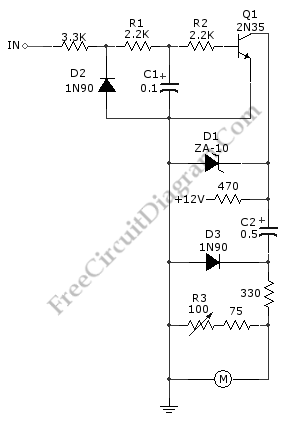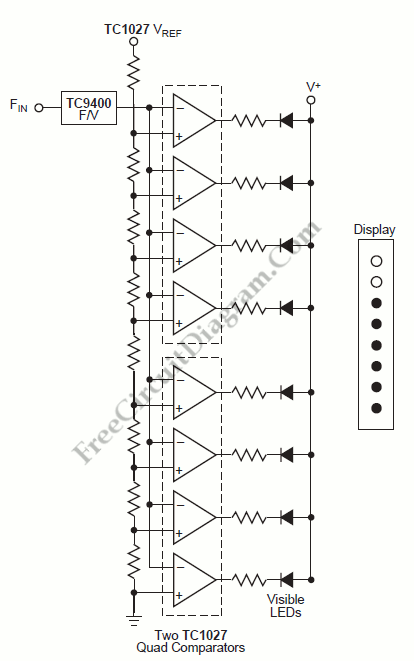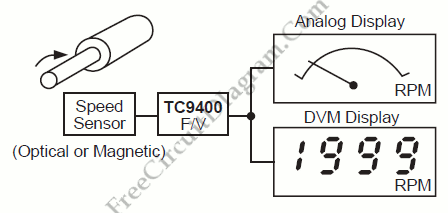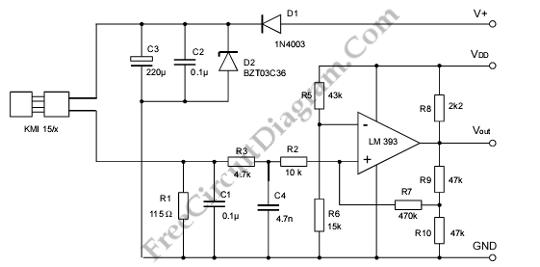## Tachometer Circuit with Single TransistorA schematic diagram of  simple tachometer circuit is shown below. Using only a single transistor as the active component, this tachometer would probably be the simplest one. The input of this circuit can be picked up from ignition pickup coil,  or other wiring that gives ignition wave-form. This tachometer circuit works for 0 to 6,000 rpm. R3 is used for […]

## F/V Converter for Tachometer Bar Graph DisplayWe can construct a tachometer using the TC9400 in the F/V mode to convert the frequency information (RPM) into a linearly proportional voltage. Then we can compare this voltage to one of “n” comparators (on this example using 8). The respective LED lights up and will continue to stay lit as long as the voltage exceeds the trip point when […]

## RPM/Speed IndicationOne example of frequency-to-voltage converter application is RPM/Speed indication, converting pulse signal from speed sensor into a linear voltage to drive analog meter. Here is the  block diagram of such speed indication system: Since they measure the number of events per time period, flow rates and revolutions per second are nothing more than frequency signals. This flow and revolution will […]